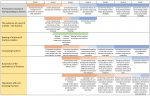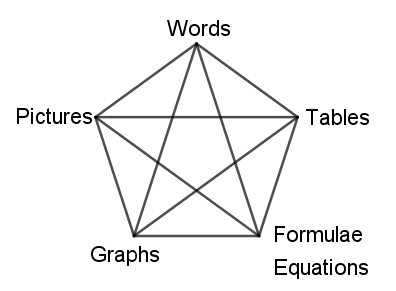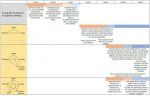• Developing the Fraction Concept (illustrative trajectory)

In this section, we illustrate the developmental trajectory for fractions. This illustration is indicative of the progression with some milestones illustrated along the way.

The NumberSense Mathematics Programme learning trajectory for the development of the fraction concept assumes that there are a number of interrelated concepts within the overarching concept of fractions. For the primary school years these are:

• The existence of a part of a whole: the fraction
• Naming of fractions and fraction notation (social knowledge)
• Comparing fractions
• Awareness of the equivalence of fractions
• Operations with and involving fractionsFraction Trajectory Illustration
• Developing Pre-Algebraic Thinking (illustrative trajectory)

In this section we illustrate the developmental trajectory for algebraic thinking. This illustration is indicative of the progression with some milestones illustrated along the way.

The NumberSense Mathematics Programme learning trajectory for the development of algebraic thinking assumes that mathematical situations can be described in at least five different ways, illustrated below.Furthermore, the learning trajectory for the development of algebraic thinking assumes that the development of robust algebraic thinking relies on an awareness of the interrelatedness of these different representations. That is, different patterns (direct proportion, linear and non-linear) have different characteristics in each representation. At the same time, the manifestation of a given characteristic in one representation can be used to explain the manifestation of that characteristic in other representations and the ability to switch between the different representations contributes to confidence with algebraic thinking and formal algebra.Algebraic Thinking Trajectory Illustration